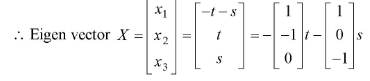# May 2019

May 19 1a)​​ If​​ X1​​ has mean 4 and variance 9 and​​ X2​​ has mean -2 and variance 4 where​​ X1 and ​​ X2​​ are independent, find​​ E2X1+X23​​ and​​ V2X1+X23 ​​ ​​ ​​ ​​ ​​ ​​ ​​ ​​ ​​ ​​​​  ​​ ​​ ​​ ​​ ​​ ​​ ​​ ​​ ​​ ​​ ​​ ​​ ​​ ​​ ​​ ​​ ​​ ​​ ​​ ​​ ​​ ​​ ​​ ​​ ​​ ​​ ​​ ​​ ​​ ​​ ​​ ​​ ​​ ​​ ​​ ​​ ​​ ​​ ​​ ​​ ​​ ​​ ​​ ​​ ​​ ​​ ​​ ​​ ​​ ​​ ​​ ​​ ​​ ​​ ​​ ​​ ​​ ​​ ​​ ​​ ​​ ​​ ​​ ​​ ​​ ​​ ​​ ​​ ​​ ​​ ​​ ​​ ​​ ​​ ​​ ​​ ​​ ​​ ​​ ​​ ​​ ​​ ​​ ​​ ​​ ​​ ​​​​  ​​​​ 5M

Given that for​​ X1​​ : Mean = 4, Variance = 9

For​​ X2​​ : Mean = -2 , Variance = 4

Since​​ X1, X2​​ are independent,

EaX+bY+c=aEX+bEY+c​​

VaX+bY+c=a2VX+b2VY​​

E2X1+X23=2EX1+EX23​​

​​ ​​ ​​ ​​​​ =24+23

​​ ​​ ​​ ​​ ​​ ​​ ​​ ​​​​ =3​​

​​ V2X1+X23=22VX1+12VX2​​

​​ ​​ ​​ ​​ ​​ ​​ ​​ ​​ ​​ ​​ ​​ ​​​​ =49+14

​​ ​​ ​​ ​​ ​​ ​​ ​​ ​​ ​​ ​​ ​​ ​​ ​​​​ =40

May 19 1b) Find the extremals of​​ x1x2x+yydx ​​ ​​ ​​ ​​ ​​ ​​ ​​ ​​ ​​ ​​ ​​ ​​ ​​ ​​ ​​ ​​ ​​ ​​ ​​ ​​ ​​ ​​ ​​ ​​ ​​ ​​ ​​ ​​ ​​ ​​ ​​ ​​ ​​ ​​ ​​ ​​ ​​ ​​ ​​ ​​ ​​ ​​ ​​ ​​ ​​ ​​ ​​ ​​ ​​ ​​ ​​ ​​ ​​​​ 5M

Let ​​ F=x+yy=xy+y2​​

Since F does not contain y explicitly, by corollary of Euler’s Lagrange equation​​ Fy=c​​

x.1+2y=c​​

2dydx=cx​​

dy=12cxdx​​

On integrating,​​ y=12cxx22+c2​​

y=12cx14x2+c2​​

Put​​ c1=12c​​ ​​

y=14x2+c1x+c2 ​​​​ is the required extremal.

May 19​​ 1b)​​ Verify​​ Cauchy-Schwartz inequality​​ u=4, 2, 1 and v=8,4,2 ​​ ​​ ​​ ​​ ​​ ​​​​  ​​ ​​ ​​ ​​ ​​ ​​ ​​ ​​ ​​ ​​ ​​ ​​ ​​ ​​ ​​ ​​ ​​ ​​ ​​ ​​ ​​ ​​ ​​ ​​ ​​ ​​ ​​ ​​ ​​ ​​ ​​ ​​ ​​ ​​ ​​ ​​ ​​ ​​ ​​ ​​ ​​ ​​ ​​ ​​ ​​ ​​ ​​ ​​ ​​ ​​ ​​ ​​ ​​ ​​ ​​ ​​ ​​ ​​ ​​ ​​ ​​ ​​ ​​ ​​ ​​ ​​ ​​ ​​ ​​ ​​ ​​ ​​ ​​ ​​ ​​ ​​ ​​ ​​ ​​ ​​ ​​ ​​ ​​ ​​ ​​ ​​ ​​ ​​ ​​ ​​ ​​ ​​ ​​ ​​ ​​ ​​ ​​ ​​ ​​ ​​ ​​ ​​ ​​ ​​ ​​​​ 5M

Given that,​​ u=4, 2, 1 and v=8,4,2

​​ u=42+22+12=21

v=82+(4)2+(2)2=84​​

Also,​​ u.v=21×84=42 ………(1)

u, v=48+24+12=3282​​

​​ ​​ ​​ ​​ ​​ ​​ ​​ ​​ ​​ ​​​​ =42​​

u, v=42​​ ………..(2)

From (1) and (2),

u, v=u.v  ​​

Thus, the​​ Cauchy-Schwartz inequality is verified.

May 19​​ 1d) Show that the matrix​​ A=223111131​​ is non-derogatory.  ​​ ​​ ​​ ​​ ​​ ​​ ​​ ​​ ​​ ​​ ​​ ​​ ​​ ​​ ​​ ​​ ​​​​ 5M

Let​​ λ​​ be Eigen Value of matrix A.

Characreistic equation is​​ Aλ=0​​

2λ2311λ1131λ=0​​

λ32+11λ2+45+4λ6=0 ​​

λ32λ25λ+6=0​​

​​ Eigen values,​​ λ​​ are 1, 2, -3

Since the eigen values of given matrix are distinct, Matrix A is non-derogatory.

May 19 2a) Using Cauchy’s​​ Residue​​ theorem evaluate​​ cz1z+12z2 ​​ where c is |z| =4  ​​ ​​ ​​ ​​ ​​ ​​ ​​ ​​​​  ​​ ​​ ​​ ​​ ​​ ​​ ​​ ​​ ​​ ​​ ​​ ​​ ​​ ​​ ​​ ​​ ​​ ​​ ​​ ​​ ​​ ​​ ​​ ​​ ​​ ​​ ​​ ​​ ​​ ​​ ​​ ​​ ​​ ​​ ​​ ​​ ​​ ​​ ​​ ​​ ​​ ​​ ​​ ​​ ​​ ​​ ​​ ​​ ​​ ​​ ​​ ​​ ​​ ​​ ​​ ​​ ​​ ​​ ​​ ​​ ​​ ​​ ​​ ​​ ​​ ​​ ​​ ​​ ​​ ​​ ​​ ​​ ​​ ​​ ​​ ​​ ​​ ​​ ​​ ​​ ​​ ​​ ​​ ​​ ​​ ​​ ​​ ​​ ​​ ​​ ​​ ​​ ​​ ​​ ​​ ​​ ​​ ​​ ​​ ​​​​ 6M

The circle |z| =4 has centre (0, 0) and radius 4.

Here,​​ z0=1​​ is a pole of order 2 and​​ z0=2​​ is​​ a simple pole.

z0=1 and z0=2​​ lies inside the circle.​​

R1​​ = Residue of f(z) at ‘z0=1​​ ‘

​​ ​​ ​​ ​​ ​​​​ =1n1! limzz0dn1dzn1zz0n×fz​​

​​ ​​​​ =121! limz1ddzz+12×z1z+12z2

​​ ​​ ​​​​ =11!limz1z2.1z1.1z22​​

​​ ​​ ​​ ​​ ​​ ​​ ​​​​ =1122=19

R2​​ = Residue of f(z) at ‘z0=2

​​ ​​ ​​ ​​ ​​ ​​​​ =limzz0 zz0fz

​​ ​​ ​​ ​​​​ =limz2z2×z1z+12z2

​​ ​​ ​​ ​​ ​​​​ =212+12

​​ ​​ ​​ ​​ ​​​​ =19​​

By Cauchy’ Residue theorem,​​ fzdz=2πi R1+R2+R3+​​

​​​​ cz1z+12z+1dz=2πi . 19+19

​​ ​​ ​​ ​​ ​​ ​​ ​​ ​​ ​​ ​​ ​​ ​​ ​​ ​​ ​​ ​​ ​​ ​​ ​​ ​​ ​​ ​​ ​​ ​​ ​​ ​​ ​​ ​​ ​​​​ =0​​

​​​​

May 19 2b) Show that the extremal of the isoperimetric problem​​ lyx=x1x2y2dx=k​​ ​​ is a parabola.  ​​ ​​ ​​ ​​ ​​ ​​ ​​ ​​ ​​ ​​ ​​ ​​ ​​ ​​ ​​ ​​ ​​ ​​ ​​​​  ​​ ​​ ​​ ​​ ​​ ​​ ​​ ​​ ​​ ​​ ​​ ​​ ​​ ​​ ​​ ​​ ​​ ​​ ​​ ​​ ​​ ​​ ​​ ​​ ​​ ​​ ​​ ​​ ​​ ​​ ​​ ​​ ​​ ​​ ​​ ​​ ​​ ​​ ​​ ​​ ​​ ​​ ​​ ​​ ​​ ​​ ​​ ​​ ​​ ​​ ​​ ​​ ​​​​ 6M ​​ ​​ ​​ ​​ ​​ ​​ ​​ ​​ ​​ ​​ ​​ ​​ ​​ ​​ ​​ ​​ ​​ ​​ ​​ ​​ ​​ ​​ ​​ ​​ ​​ ​​ ​​ ​​ ​​ ​​ ​​ ​​ ​​ ​​ ​​ ​​ ​​ ​​ ​​ ​​ ​​ ​​ ​​ ​​ ​​ ​​ ​​ ​​ ​​ ​​ ​​ ​​ ​​ ​​ ​​ ​​ ​​ ​​ ​​ ​​ ​​ ​​ ​​ ​​ ​​ ​​ ​​ ​​ ​​ ​​​​  ​​ ​​ ​​ ​​ ​​ ​​ ​​ ​​ ​​ ​​ ​​ ​​ ​​ ​​ ​​ ​​ ​​ ​​ ​​ ​​ ​​ ​​ ​​ ​​ ​​ ​​ ​​ ​​​​

Let ​​ x1x2F dx=x1x2y2dx​​ and​​ x1x2G dx=x1x2y dx=k

F=y2  and G=y​​

Therefore, Lagrangian function,​​ H=F+λG​​

H=y2+λy​​ ………………..(1)

Hy=2y​​ …………….(2)

and​​ x1x2H dx=x1x2y2+λydx

Since the above integral does not contain ‘x’ explicitly, by corollary of Euler’s Lagrange equation,​​ HyHy=c

y2+λyy.2y=c​​ ………from (1) and (2)

y2+λy=c​​

y2=λyc​​

y=dydx=λyc​​

1λycdy=dx​​

1y ycλdy=dx​​

1λycλ12dy=dx​​

On integrating,​​ 1λ.ycλ1212=x+c1

ycλ12=λ2x+c1​​

Squaring both sides,

ycλ=λ4x+c12​​

y=λ4x+c12+cλ ​​​​ which is a parabola.

May 19 4c) Is the matrix​​ A=211121001​​ diagonalisable? If so find the diagonal matrix and the transforming matrix.  ​​ ​​ ​​ ​​ ​​ ​​ ​​ ​​ ​​ ​​ ​​ ​​ ​​ ​​ ​​ ​​ ​​ ​​ ​​ ​​ ​​ ​​ ​​ ​​ ​​ ​​ ​​ ​​ ​​ ​​ ​​ ​​ ​​ ​​ ​​ ​​ ​​ ​​ ​​ ​​ ​​​​ 8M

Let​​ λ​​ be the eigen vector of matrix A.

Characteristic equation is,​​ AλI=0​​

2λ1112λ1001λ=0​​

λ32+2+1λ2+2+2+3λ=0​​

λ35λ2+7λ3=0​​

Thus, the Eigen values are 1, 1, 3

I: When​​ λ=1​​

AλIX=0​​

111111000.x1x2x3=000​​

R2R1​​

111000000.x1x2x3=000​​ …………..(1)

No. of unknowns (n) =3

Rank ( r)= no. of non-zero rows =1

Algebraic multiplicity (A. M) = No. of times ‘λ=1’ is repeated =2​​

Geometric multiplicity (G. M) = n-r = 3 – 1 = 2

​​ A. M = G. M for ​​ ‘λ=1

Expanding (1),

x1+x2+x3=0​​

Put​​ x2=t and x3=s​​

x1+t+s=0​​

x1=ts​​Therefore, Eigen Vector X​​ =x1x2x3=tsts=110t101s

Eigen vector ​​ X1=1 1   0 and X2=1  0 1​​

II: When​​ λ=3

AλIX=0​​

111111002.x1x2x3=000​​

R2+R1​​

111002002.x1x2x3=000​​

R3+R2;0.5R2​​

111001000.x1x2x3=000​​ …………(2)

Rank ( r) = 2

A.M = No. of time ‘λ=3’ is repeated = 1

G.M = n-r =3-2 =1

​​ A. M =G. M ​​ for​​ λ=3

Expanding (2)

x3=0​​

x1+x2+x3=0​​

x1+x2+0=0​​

x1=x2​​

Therefore, Eigen vector X​​ =x1x2x3=x2x20=110x2

Eigen vector​​ X3= 1 1 0

Since, A. M = G. M for all eigen values, matrix A is diagonalizable.

M1AM=D​​

Thus, Matrix A is diagonalized to diagonal matrix D by the transforming Matrix M, where

D=100010003​​ and​​ M=011101110

Tab Content
Tab Content
Tab Content
Tab Content
Tab Content
Tab Content
Tab Content
Tab Content
Tab Content
Tab Content
Tab Content

May 19 6c) For the following data

 X 100 110 120 130 140 150 160 170 180 190 Y 45 51 54 61 66 70 74 78 85 89

Find the coefficients of regression​​ bxy  and byx​​ and the coefficient of correlation ( r)  ​​ ​​ ​​ ​​ ​​ ​​ ​​ ​​ ​​ ​​ ​​ ​​ ​​ ​​ ​​ ​​ ​​​​  ​​ ​​ ​​ ​​ ​​ ​​ ​​ ​​ ​​ ​​ ​​ ​​ ​​ ​​ ​​ ​​ ​​ ​​ ​​ ​​ ​​ ​​ ​​ ​​ ​​ ​​ ​​ ​​ ​​ ​​ ​​ ​​ ​​ ​​ ​​ ​​ ​​ ​​ ​​ ​​ ​​ ​​ ​​ ​​ ​​ ​​ ​​ ​​ ​​ ​​ ​​ ​​ ​​ ​​ ​​ ​​ ​​ ​​ ​​ ​​ ​​ ​​ ​​ ​​ ​​ ​​ ​​ ​​ ​​ ​​ ​​ ​​ ​​ ​​ ​​ ​​ ​​ ​​ ​​ ​​ ​​ ​​ ​​ ​​ ​​ ​​ ​​ ​​ ​​ ​​ ​​ ​​ ​​ ​​ ​​ ​​​​ 8M

Let a = 145, b = 67, c =10, c’​​ = 1, n​​ = 4

 X Y u = X – 145 V = Y- 67 u2​​ u v v2​​ 100 45 -45 -22 2025 900 484 110 51 -35 -16 1225 560 256 120 54 -25 -13 625 325 169 130 61 -15 -6 225 90 36 140 66 -5 -1 25 5 1 150 70 5 3 25 15 9 160 74 15 7 225 105 225 170 78 25 11 625 275 625 180 85 35 18 1225 630 324 190 89 45 22 2025 990 484 Total 0 3 8250 3985 1933

Here n= 10

x=a+cu+a+c.un=145+010=145​​

y=b+cv=b+c.vn=67+1.310=67.3​​

byx=bvu=nuvu v nu2u2=1039850310825002=0.4830​​

bxy=buv=nuvu v nv2v2=1039850310193332=2.0616​​

Coefficient of correlation is​​ given by,

rx,y=byx×bxy​​

​​ ​​ ​​ ​​ ​​ ​​ ​​ ​​ ​​​​ =0.4830×2.0616

​​ ​​ ​​ ​​ ​​ ​​ ​​​​ =0.9979​​